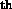Next: Introduction Up: No Title Previous: Calculating the Equation of

# Numerical Methods

The equations of motion derived in the previous chapter (equation (3.35)) have a strongly non-linear term, and is therefore practically impossible to solve analytically. The only way of finding the galaxy orbits is to make use of one of the big wonders of the 20century; numerical analysis using computers. Section 4.1 serves as an introduction to the concept of optimization, Section 4.2 deals with numerical integration, and Section 4.3 presents the optimizing methods with the different implementations that are considered in this thesis.

Trond Hjorteland
Mon Jul 5 02:59:28 MET DST 1999